Next: 5.2 Field Calculation over Up: 5.1 Exposure Kinetics Previous: 5.1.3 Modeling of Chemically

## 5.1.4 Simulation Flow

The preceding discussion shows that for conventional as well as for chemically amplified resists the fundamental interaction between the resist material and the exposing light is modeled by the simple first-order dissolution laws of (5.12) and (5.15), i.e.,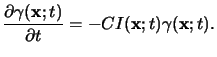(5.18)

Here the quantity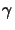(x;t) stands vicariously for the PAC m(x;t) and the PAG g(x;t) depending on the respective resist type. The optical properties, e.g., the refractive index n(x;t) of the resist is determined by (5.3) and (5.8), which combines to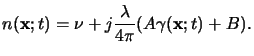(5.19)

The two coupled equations (5.19) and (5.20) are the central relations of the exposure/bleaching module and were first proposed by Frederick Dill and co-workers in his famous paper series [135,74,140,141].

As can be seen from (5.19) the absorbed light intensity I(x;t) inside the optically nonlinear resist has to be determined. Because the bleaching rate of(x;t) is almost negligible when compared to the frequency of the EM field, the refractive index n(x;t) varies only slowly with respect to the field propagation and thus a quasi-static approximation can be applied to (5.19), i.e.,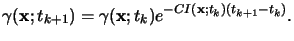(5.20)

For the same reason a steady-state field distribution can be assumed within a time-step tk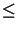t < tk + 1. Hence the time-dependence of the EM field is a harmonic one and can be described most conveniently by a phasor notation like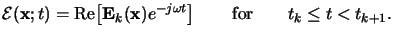(5.21)

This means that the field phasor Ek(x) for a certain time-step tk has to be calculated in an inhomogeneous medium with a spatially varying permittivity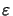(x;tk). The permittivity itself is related to the refractive index by Maxwell's formula [11, p. 13],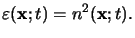(5.22)

Once the field phasor Ek(x) is determined the absorbed light intensity is easily calculated from it,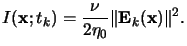(5.23)

Now I(x;tk) can simply be inserted into (5.21) to obtain the chemical state(x;tk + 1) of the photoresist for the next time step tk + 1.

This procedure is graphically illustrated in the simulation flow of Figure 5.1. The crucial step throughout is the calculation of the EM field phasor Ek(x) in (5.22). Depending on the application, the required accuracy, and the dimensionality of the simulation various methods exist. They range from simple vertical scalar models to the physically most rigorous approach based on a direct solution of the Maxwell equations. A survey over the most important simulation methods is presented in the subsequent two sections, whereas in the next chapter a newly developed rigorous three-dimensional approach is described.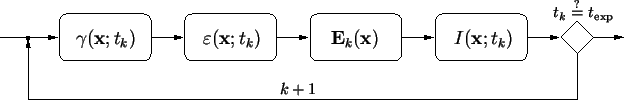Before we begin with the discussion of field calculation, we want to point out an important property of the quasi-static approximation applied above. Principally the resist is an optically nonlinear medium. Because of the different time constants, i.e., the field varies much faster than the chemical processes occur, the dissolution law (5.19) can be explicitly discretized with respect to time (cf. (5.21)) and the time-dependence of the field can be assumed to be a harmonic one (cf. (5.22)). Hence the nonlinear time-varying problem is transformed to a series of inhomogeneous linear steady-state problems.Next: 5.2 Field Calculation over Up: 5.1 Exposure Kinetics Previous: 5.1.3 Modeling of Chemically
Heinrich Kirchauer, Institute for Microelectronics, TU Vienna
1998-04-17